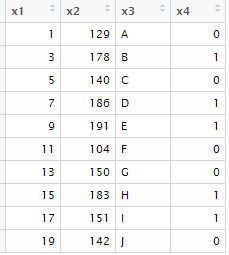# R : If Else and Nested If Else

The If-Else statements are important part of R programming. In this tutorial, we will see various ways to apply conditional statements (If..Else nested IF) in R. In R, there are a lot of powerful packages for data manipulation. In the later part of this tutorial, we will see how IF ELSE statements are used in popular packages.

Sample Data

Let's create a sample data to show how to perform IF ELSE function. This data frame would be used further in examples.
x1 x2 x3
1 129 A
3 178 B
5 140 C
7 186 D
9 191 E
11 104 F
13 150 G
15 183 H
17 151 I
19 142 J

Run the program below to generate the above table in R.
```set.seed(123)
mydata = data.frame(x1 = seq(1,20,by=2),
x2 = sample(100:200,10,FALSE),
x3 = LETTERS[1:10])
```
x1 = seq(1,20,by=2) : The variable 'x1' contains alternate numbers starting from 1 to 20. In total, these are 10 numeric values.

x2 = sample(100:200,10,FALSE) : The variable 'x2' constitutes 10 non-repeating random numbers ranging between 100 and 200.

x3 = LETTERS[1:10] : The variable 'x3' contains 10 alphabets starting from A to Z.

Syntax of ifelse() function :

The ifelse() function in R works similar to MS Excel IF function. See the syntax below -
ifelse(condition, value if condition is true, value if condition is false)

## Example 1 : Simple IF ELSE Statement

Suppose you are asked to create a binary variable - 1 or 0 based on the variable 'x2'. If value of a variable 'x2' is greater than 150, assign 1 else 0.
mydata\$x4 = ifelse(mydata\$x2>150,1,0)
In this case, it creates a variable x4 on the same data frame 'mydata'. The output is shown in the image below -ifelse : Output
Create variable in a new data frame
Suppose you need to add the above created binary variable in a new data frame. You can do it by using the code below -
```x = ifelse(mydata\$x2>150,1,0)
newdata = cbind(x,mydata)
```
The cbind() is used to combine two vectors, matrices or data frames by columns.

Apply ifelse() on Character Variables
If variable 'x3' contains character values - 'A', 'D', the variable 'x1' should be multiplied by 2. Otherwise it should be multiplied by 3.
mydata\$y = ifelse(mydata\$x3 %in% c("A","D") ,mydata\$x1*2,mydata\$x1*3)
The output is shown in the table below
```x1 x2 x3 y
1 129 A 2
3 178 B 9
5 140 C 15
7 186 D 14
9 191 E 27
11 104 F 33
13 150 G 39
15 183 H 45
17 151 I 51
19 142 J 57
```

## Example 2 : Nested If ELSE Statement in R

Multiple If Else statements can be written similarly to excel's If function. In this case, we are telling R to multiply variable x1 by 2 if variable x3 contains values 'A' 'B'. If values are 'C' 'D', multiply it by 3. Else multiply it by 4.
```mydata\$y = ifelse(mydata\$x3 %in% c("A","B") ,mydata\$x1*2,
ifelse(mydata\$x3 %in% c("C","D"), mydata\$x1*3,
mydata\$x1*4))
```
Do you hate specifying data frame multiple times with each variable?

You can use with() function to avoid mentioning data frame each time. It makes writing R code faster.
```mydata\$y = with(mydata, ifelse(x3 %in% c("A","B") , x1*2,
ifelse(x3 %in% c("C","D"), x1*3, x1*4)))
```

## Special Topics related to IF ELSE

In this section, we will cover the following topics -
1. How to treat missing (NA) values in IF ELSE.
2. How to use OR and AND operators in IF ELSE
3. Aggregate or Summary Functions and IF ELSE Statement

Handle Missing Values

Incorrect Method
x = NA
ifelse(x==NA,1,0)
Result :  NA
It should have returned 1.

Correct Method
x = NA
ifelse(is.na(x),1,0)
Result : 1
The is.na() function tests whether a value is NA or not.

Use OR and AND Operators

The & symbol is used to perform AND conditions
ifelse(mydata\$x1<10 & mydata\$x2>150,1,0)
Result : 0 1 0 1 1 0 0 0 0 0

The | symbol is used to perform OR conditions
ifelse(mydata\$x1<10 | mydata\$x2>150,1,0)
Result : 1 1 1 1 1 0 0 1 1 0

Count cases where condition meets

In this example, we can counting the number of records where the condition meets.
sum(ifelse(mydata\$x1<10 | mydata\$x2>150,1,0))
Result : 7

If Else Statement : Another Style

There is one more way to define if..else statement in R. This style of writing If Else is mostly used when we use conditional statements in loop and R functions. In other words, it is used when we need to perform various actions based on a condition.

Syntax -
if(condition) yes else no
k = 99
if(k > 100) 1 else 0
Result : 0
If..Else If..Else Statements
k = 100
if(k > 100){
print("Greater than 100")
} else if (k < 100){
print("Less than 100")
} else {
print ("Equal to 100")
}
Result : "Equal to 100"

### If Else in Popular Packages

1. dplyr package

if_else(condition, value if condition is true, value if condition is false, value if NA)

The following program checks whether a value is a multiple of 2
```library(dplyr)
x=c(1,NA,2,3)
if_else(x%%2==0, "Multiple of 2", "Not a multiple of 2", "Missing")
```
```Result :
Not a multiple of 2
Missing
Multiple of 2
Not a multiple of 2
```
The %% symbol returns remainder after a value is divided by divisor. In this case, first element 1 is divided by 2.

2. sqldf package
We can write SQL query in R using sqldf package. In SQL, If Else statement is defined in CASE WHEN.
```df=data.frame(k=c(2,NA,3,4,5))
library(sqldf)
sqldf(
"SELECT *,
CASE WHEN (k%2)=0  THEN 'Multiple of 2'
WHEN  k is NULL  THEN 'Missing'
ELSE 'Not a multiple of 2'
END AS T
FROM df"
)
```
```Output

k T
2 Multiple of 2
NA Missing
3 Not a multiple of 2
4 Multiple of 2
5 Not a multiple of 2
```Share
Related Posts1.2.